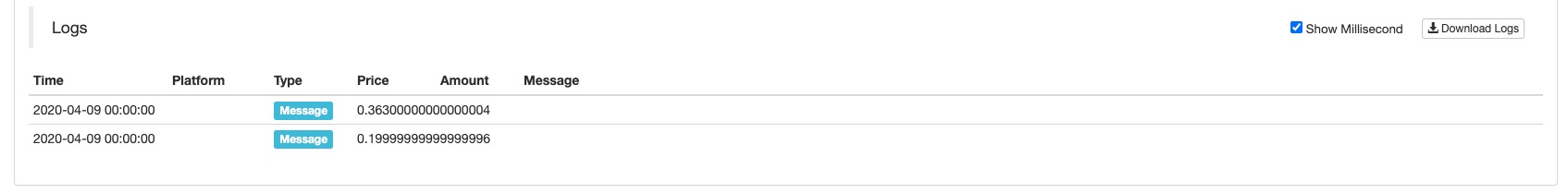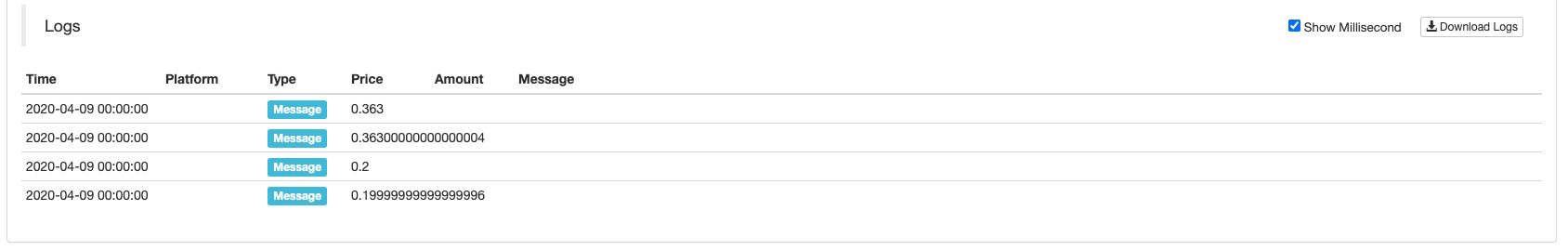# Solution of numerical calculation accuracy problem in JavaScript strategy design

Author: , Created: 2020-07-09 11:31:01, Updated:

When writing JavaScript strategies, due to some problems of the scripting language itself, it often leads to numerical accuracy problems in calculations. It has a certain influence on some calculations and logical judgments in the program. For example, when calculating `1 - 0.8` or `0.33 * 1.1`, the error data will be calculated:

``````function main() {
var a = 1 - 0.8
Log(a)

var c = 0.33 * 1.1
Log(c)
}
``````So how to solve such problems? The root of the problem is:

The maximum progress of floating-point values is 17 decimal places, but the accuracy is far worse than integers when performing operations; integers are converted to decimal when performing operations; and when calculating decimal operations in Java and JavaScript, both First convert the decimal decimal to the corresponding binary, part of the decimal can not be completely converted to binary, here is the first error. After the decimals are converted to binary, then the operation between the binary is performed to obtain the binary result. Then convert the binary result to decimal, where a second error usually occurs.

In order to solve this problem, I searched for some solutions on the Internet, and tested and used the following solutions to solve this problem:

``````function mathService() {
var c, d, e;
try {
c = a.toString().split(".").length;   // Get the decimal length of a
} catch (f) {
c = 0;
}
try {
d = b.toString().split(".").length;   // Get the decimal length of b
} catch (f) {
d = 0;
}
//Find e first, multiply both a and b by e to convert to integer addition, then divide by e to restore
return e = Math.pow(10, Math.max(c, d)), (this.mul(a, e) + this.mul(b, e)) / e;
}

// multiplication
this.mul = function(a, b) {
var c = 0,
d = a.toString(),         // Convert to string
e = b.toString();         // ...
try {
c += d.split(".").length;      // c Accumulate the fractional digit length of a
} catch (f) {}
try {
c += e.split(".").length;      // c Accumulate the length of decimal places of b
} catch (f) {}
// Convert to integer multiplication, then divide by 10^c, move decimal point, restore, use integer multiplication without losing accuracy
return Number(d.replace(".", "")) * Number(e.replace(".", "")) / Math.pow(10, c);
}

// Subtraction
this.sub = function(a, b) {
var c, d, e;
try {
c = a.toString().split(".").length;  // Get the decimal length of a
} catch (f) {
c = 0;
}
try {
d = b.toString().split(".").length;  // Get the decimal length of b
} catch (f) {
d = 0;
}
return e = Math.pow(10, Math.max(c, d)), (this.mul(a, e) - this.mul(b, e)) / e;
}

// Division
this.div = function(a, b) {
var c, d, e = 0,
f = 0;
try {
e = a.toString().split(".").length;
} catch (g) {}
try {
f = b.toString().split(".").length;
} catch (g) {}
// Similarly, convert to integer, after operation, restore
return c = Number(a.toString().replace(".", "")), d = Number(b.toString().replace(".", "")), this.mul(c / d, Math.pow(10, f - e));
}
}

function main() {
var obj = new mathService()

var a = 1 - 0.8
Log(a)

var b = obj.sub(1, 0.8)
Log(b)

var c = 0.33 * 1.1
Log(c)

var d = obj.mul(0.33, 1.1)
Log(d)
}
``````The principle is to convert the two operands to be calculated into integers to calculate to avoid accuracy problems. After calculation (according to the magnification when converted to integers), the calculation results are restored to obtain accurate results.

In this way, when we want the program to place an order when the market price plus a minimum price precision, we don’t have to worry about numerical accuracy

``````function mathService() {
....    // Omitted
}

function main() {
var obj = new mathService()
var depth = exchange.GetDepth()